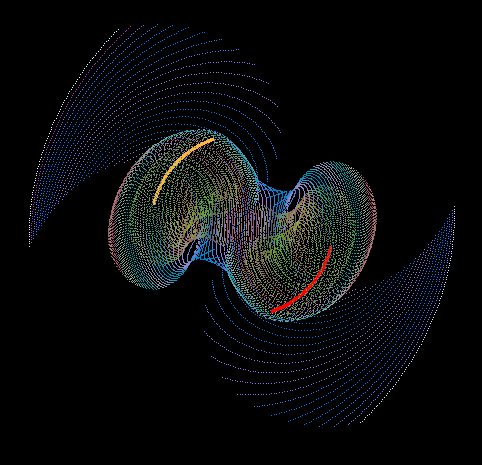This Data Model displays how Galactical Rotation Curves in Spiral Galaxies are formed due to twin centers of gravity. The Yellow and Red lines are the movements of a pair of white holes: Supermassive bodies that emit mass and energy that then manifest as stars. The other lines are the movements of the stars themselves. Note how the green lines rpresent stars that are being flung out by the slingshot effect at a faster rate than the movements of the white holes and the stars nearby the white holes. There are a huge variety of potential orbits in a binary system.

.

 Click the link below and Download Orbit Game 4.3 (August 2014) >> orbit-game-4-3.exe << If your PC is set to use the comma as a decimal separator, then this app won't work so good. orbit-game-4-3.exe demonstrates how the binary pair move away from each other as they lose mass due to stars having being emitted. Thus stars emitted subsequently are effected by a lesser gravitational force, moving them more slowly. The outer and older stars have often reached escape velocity.This model represnts the variety of potential orbits in a binary system. Stars that pass between the supermassive white holes are at their slowest in the dead center, explaining why there is little or no velocity at this point. These stars collide with each other, which then gives off the characteristic light bulge at the center of the spiral galaxy.

 Latest Article: Gravitational Waves & General Relativity

 In order to generate this software, some other questions needed to be answered: Xeno's Paradox, which deals with the math of Max Planck and quantum time, Quantum Gravity, which explains the slingshot affect, and The Many-Body-Problem, which opens a myriad of other possibilities. Gravity, a summary which includes other calculations. Summary of Rotation Curves of Galaxies, describes the nature of how dark matter does not affect Newton's law of gravity

< top >

< seti >

< why? >

< gravity >

< dark matter >

< dark energy >

< binary orbits >

< the big unwind >

< force of gravity >

< rubin's problem >

< zeno and planck >

< quantum gravity >

< relativity revised >

< gravity simulators >

< entothopter videos >

< gravitational lensing >

< the many-body problem >

< how the solar system formed >

< gravitational waves & general relativity >

< why the moon is receding from the earth >

< why the moon is red during a lunar eclipse >

< oscillations in rotation curves of spiral galaxies >

< more calculations of rotation curves of galaxies >